# 四维博客－ʩ# 四维博客－ʩ

### 初升高数学摸底测试

posted on 2018-07-18 12:53:22 | under 数学 |
1. 下列运算正确的是 $(\qquad)$

A. $a^2\cdot a^3=a^6$

B. $(ab)^2=a^2b^2$

C. $(a^2)^3=a^5$

D. $a^2+a^2=a^4$

2. 对于实数 $x$ ，我们规定 $\lfloor x\rfloor$ 表示不大于 $x$ 的最大整数，例如 $\lfloor1.2\rfloor=1$ ， $\lfloor3\rfloor=3$ ， $\lfloor-2.5\rfloor=-3$ 。若 $\displaystyle\left\lfloor\frac{x+4}{10}\right\rfloor=5$ ，则 $x$ 的取值可以是 $(\qquad)$

A. $40$

B. $45$

C. $51$

D. $56$

3. $A$ 、 $B$ 两地相距 $160$ 千米，甲车和乙车的平均速度之比为 $4:5$ ，两车同时从 $A$ 地出发到 $B$ 地，乙车比甲车早到 $30$ 分钟。若求甲车的平均速度，设甲车平均速度为 $4x$ 千米／时，则所列方程是 $(\qquad)$

A. $\displaystyle\frac{160}{4x}-\frac{160}{5x}=30$

B. $\displaystyle\frac{160}{4x}-\frac{160}{5x}=\frac12$

C. $\displaystyle\frac{160}{5x}-\frac{160}{4x}=\frac12$

D. $\displaystyle\frac{160}{4x}+\frac{160}{5x}=30$

4. 已知关于 $x$ 的方程 $x^2-(k+2)x+2k+1=0$ 的俩实数根为 $x_1^{}$ 、 $x_2^{}$ ，若 $x_1^2+x_2^2=11$ ，则实数 $k$ 的值为 $(\qquad)$

A. $-3$

B. $3$

C. $±3$

D. 无解

5. 如图所示， $AB$ 是 $\odot O$ 的切线， $B$ 为切点， $AC$ 经过点 $O$ ，与 $\odot O$ 分别相交于点 $D$ 、 $C$ 。若 $∠ACB=30\degree$ ， $AB=\sqrt3$ ，则阴影部分的面积是 $(\qquad)$A. $\displaystyle\frac{\sqrt3}2$

B. $\displaystyle\fracπ6$

C. $\displaystyle\frac{\sqrt3}2-\fracπ6$

D. $\displaystyle\frac{\sqrt3}3-\fracπ6$

6. 二次函数 $y=ax^2+bx+c$ 的图像如图所示，反比例函数 $\displaystyle y=\frac{ac}x$ 与正比例函数 $y=bx$ 在同一坐标系内的大致图像是 $(\qquad)$A.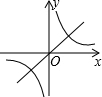B.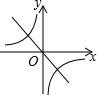C.D.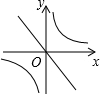7. 如图，已知点 $A\;(-8,0)$ 和点 $B\;(2,0)$ ，点 $C$ 在直线 $\displaystyle y=-\frac34x+4$ 上，则使 $\triangle ABC$ 是直角三角形的点 $C$ 的个数为 $(\qquad)$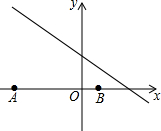A. $1$

B. $2$

C. $3$

D. $4$

## 解析：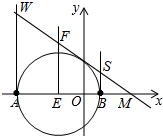如图，

① 当 $∠A$ 为直角时，过点 $A$ 作垂线与直线交于 $W\;(-8,10)$ ；

② 当 $∠B$ 为直角时，过点 $B$ 作垂线与直线交于 $S\;(2,2.5)$ ；

③ 当 $∠C$ 为直角时，则点 $C$ 在以线段 $AB$ 为直径、 $AB$ 中点 $E\;(-3,0)$ 为圆心的圆与直线 $\displaystyle y=-\frac34x+4$ 的交点上。

过点 $E$ 作垂线与直线交于 $\displaystyle F\left(-3,\frac{25}4\right)$ ，则 $\displaystyle EF=\frac{25}4$ 。

$∵$ 直线 $\displaystyle y=-\frac34x+4$ 与 $x$ 轴的交点 $M$ 为 $\displaystyle\left(\frac{16}3,0\right)$ ，

$∴\displaystyle EM=\frac{25}3$ ， $\displaystyle EF=\sqrt{\left(\frac{16}3+3\right)^2+\left(0-\frac{25}4\right)^2}=\frac{125}{12}$ 。

$∵E$ 到直线 $\displaystyle y=-\frac34x+4$ 的距离 $\displaystyle d=\frac{\displaystyle\frac{25}3×\frac{25}4}{\displaystyle\frac{125}{12}}=5$ ，

$∴$ 以线段 $AB$ 为直径、 $E\;(-3,0)$ 为圆心的圆与直线 $\displaystyle y=-\frac34x+4$ 恰好有一个交点，

$∴$ 直线 $\displaystyle y=-\frac34x+4$ 上有一点 $C$ 满足 $∠C=90\degree$ 。

综上所述，使 $\triangle ABC$ 是直角三角形的点 $C$ 的个数为 $3$ 。

8. 如图， $AC=BC$ ， $∠ACB=90\degree$ ，点 $D$ 在边 $BC$ 上 $($ 与 $B$ 、 $C$ 不重合 $)$ ，四边形 $ADEF$ 为正方形，过点 $F$ 作 $FG\bot AC$ ，交 $CA$ 的延长线于点 $G$ ，连接 $BF$ ，交 $DE$ 于点 $Q$ 。给出以下结论：① $AC=FG$ ；② $S_{\triangle BAF}^{}:S_{\text{\tiny矩形}BCGF}^{}=1:2$ ；③ $∠ABC=∠ABF$ ；④ $AD^2=AC\cdot FQ$ 。其中正确结论的个数是 $(\qquad)$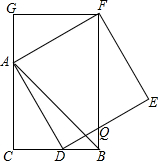A. $1$

B. $2$

C. $3$

D. $4$

## 解析：

$∵$ 四边形 $ADEF$ 为正方形，

$∴∠FAD=90\degree$ ， $AD=AF=EF$ ，

$∴∠CAD+∠FAG=90\degree$ ，

$∵FG\bot CA$ ，

$∴∠C=90\degree=∠ACB$ ，

$∴∠CAD=∠AFG$ ，

在 $\triangle FGA$ 和 $\triangle ACD$ 中， $\begin{cases}∠G=∠C\\∠AFG=∠CAD\\AF=AD\end{cases}$ ，

$∴\triangle FGA\cong\triangle ACD\;(AAS)$ ，

$∴AC=FG$ ，①正确；

$∵BC=AC$ ，

$∴FG=BC$ ，

$∵∠ACB=90\degree$ ， $FG\bot CA$ ，

$∴FG\:\!/\!\!/\:\!BC$ ，

$∴$ 四边形 $CBFG$ 是矩形，

$∴∠CBF=90\degree$ ， $\displaystyle S_{\triangle FAB}^{}=\frac12FB\cdot FG=\frac12S_{\text{\tiny四边形}CEFG}^{}$ ，②正确；

$∵CA=CB$ ， $∠C=∠CBF=90\degree$ ，

$∴∠ABC=∠ABF=45\degree$ ，③正确；

$∵∠FQE=∠DQB=∠ADC$ ， $∠E=∠C=90\degree$ ，

$∴\triangle ACD∽\triangle FEQ$ ，

$∴AC:AD=FE:FQ$ ，

$∴AD\cdot FE=AD^2=FQ\cdot AC$ ，④正确。

9. 如图，抛物线 $\displaystyle y=-\frac1{12}x^2+\frac23x+\frac53$ 与 $x$ 轴交于 $A$ 、 $B$ 两点，与 $y$ 轴交于点 $C$ 。若点 $P$ 是线段 $AC$ 上方的抛物线上的一动点，当 $\triangle ACP$ 的面积取得最大值时，点 $P$ 的坐标是 $(\qquad)$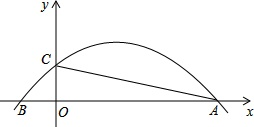A. $(4,3)$

B. $\displaystyle\left(5,\frac{35}{12}\right)$

C. $\displaystyle\left(4,\frac{35}{12}\right)$

D. $(5,3)$

## 解析：

连结 $PC$ 、 $PO$ 、 $PA$ ，设点 $P$ 的坐标为 $\displaystyle\left(m,-\frac1{12}m^2+\frac23m+\frac53\right)$ 。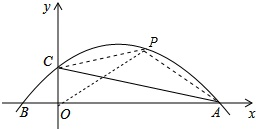令 $x=0$ ，则 $\displaystyle y=\frac53$ ，点 $C$ 的坐标为 $\displaystyle\left(0,\frac53\right)$ ，

令 $y=0$ ，则 $\displaystyle-\frac1{12}x^2+\frac23x+\frac53=0$ ，解得 $x_1^{}=-2,\;x_2^{}=10$ ，

$∴$ 点 $A$ 的坐标为 $(10,0)$ ，点 $B$ 的坐标为 $(-2,0)$ ， \displaystyle\begin{aligned}S_{\triangle PCA}^{}&=S_{\triangle PCO}^{}+S_{\triangle POA}^{}-S_{\triangle AOC}^{}\\&=\frac12×\frac53×m+\frac12×10×\left(-\frac1{12}m^2+\frac23m+\frac53\right)-\frac12×\frac53×10\\&=-\frac5{12}(m-5)^2+\frac{125}{12}\;\footnotesize\text{，}\end{aligned}

$∴$ 当 $x=5$ 时， $\triangle PAC$ 面积的最大值为 $\displaystyle\frac{125}{12}$ ，此时点 $P$ 的坐标为 $\displaystyle\left(5,\frac{35}{12}\right)$ 。

10. 已知直线 $y=-\sqrt3x+3$ 与坐标轴分别交于点 $A$ 、 $B$ ，点 $P$ 在抛物线 $\displaystyle y=-\frac13\left(x-\sqrt3\right)^2+4$ 上，能使 $\triangle ABP$ 为等腰三角形的点 $P$ 的个数为 $(\qquad)$

A. $3$

B. $4$

C. $5$

D. $6$

## 解析：

以点 $B$ 为圆心、线段 $AB$ 为半径作圆，交抛物线于点 $C$ 、 $M$ 、 $N$ ，连结 $AC$ 、 $BC$ 。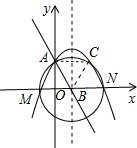令 $y=-\sqrt3x+3$ 中的 $x=0$ ，则 $y=3$ ，

$∴$ 点 $A$ 的坐标为 $(0,3)$ ；

令 $y=-\sqrt3x+3$ 中的 $y=0$ ，则 $x=\sqrt3$ ，

$∴$ 点 $B$ 的坐标为 $\left(\sqrt3,0\right)$ ，

$∴AB=2\sqrt3$ 。

$∵$ 抛物线的对称轴为 $x=\sqrt3$ ，

$∴$ 点 $C$ 的坐标为 $\left(2\sqrt3,3\right)$ ，

$∴AC=2\sqrt3=AB=BC$ ，

$∴\triangle ABC$ 是等边三角形。

令 $\displaystyle y=-\frac13\left(x-\sqrt3\right)^2+4$ 中的 $y=0$ ，则 $\displaystyle-\frac13\left(x-\sqrt3\right)^2+4=0$ ，解得 $x_1^{}=-\sqrt3,\;x_2^{}=3\sqrt3$ ，

$∴$ 点 $M$ 的坐标为 $\left(-\sqrt3,0\right)$ ，点 $N$ 的坐标为 $\left(3\sqrt3,0\right)$ 。

若 $\triangle ABP$ 是等腰三角形：

① 当 $AB=BP$ 时，以点 $B$ 为圆心、 $AB$ 为半径作圆，与抛物线交于 $C$ 、 $M$ 、 $N$ 三点；

② 当 $AB=AP$ 时，以点 $A$ 为圆心、 $AB$ 为半径作圆，与抛物线交于 $C$ 、 $M$ 两点；

③ 当 $AP=BP$ 时，作线段 $AB$ 的垂直平分线，与抛物线交于 $C$ 、 $M$ 两点；

综上所述，能使 $\triangle ABP$ 为等腰三角形的点 $P$ 的个数为 $3$ 。